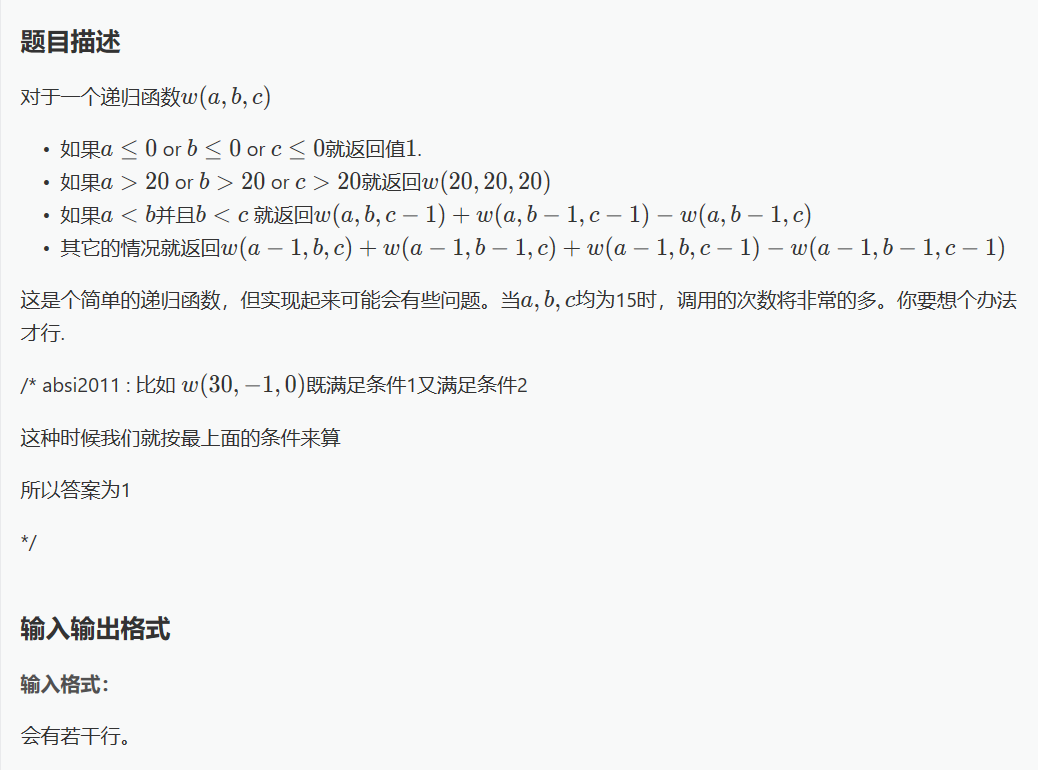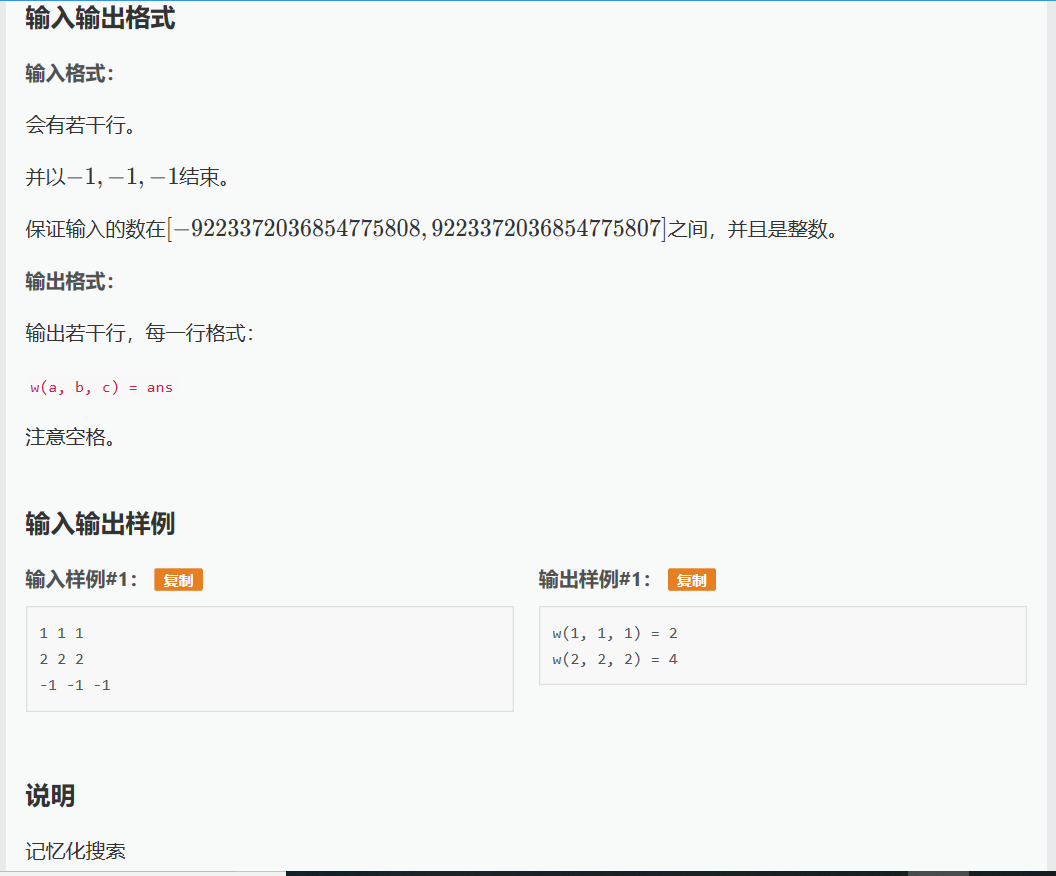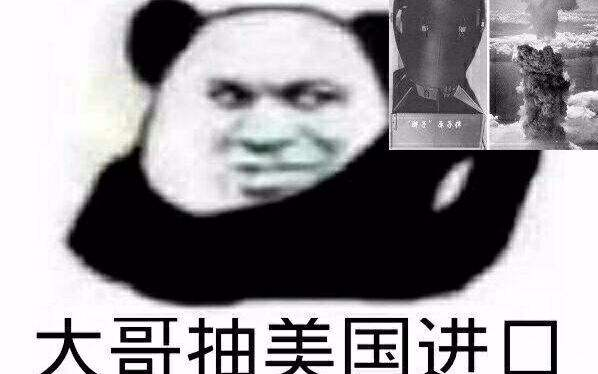#include<stdio.h>

int x;

void pai()
{
int i,j,k;
for(i=0;i<21;i++)
{
for(j=0;j<21;j++)
{
for(k=0;k<21;k++)
x[i][j][k]=10000;
}
}
for(i=0;i<21;i++)
{
for(j=0;j<21;j++)
{
x[i][j]=1;
x[i][j]=1;
x[i][j]=1;
}
}
}

int w(long long int a,long long int b,long long int c);

int main()
{
long long int a,b,c;
pai();
while(scanf("%lld%lld%lld",&a,&b,&c)!=EOF)
{
if(a==-1&&b==-1&&c==-1)
break;
else
{
printf("w(%lld, %lld, %lld)=%d\n",a,b,c,w(a,b,c));
}
}
}

int w(long long int a,long long int b,long long int c)
{
if(a<=0||b<=0||c<=0)
return x;
else if(a>20||b>20||c>20)
{
a=b=c=20;
x[a][b][c]=w(a,b,c);
return w(a,b,c);
}
else if(a<b&&b<c)
{
if(x[a][b][c]==10000)
{
x[a][b][c]=w(a,b,c-1)+w(a,b-1,c-1)-w(a,b-1,c);
}
return x[a][b][c];
}
else
{
if(x[a][b][c]==10000)
{
x[a][b][c]=w(a-1,b,c)+w(a-1,b-1,c)+w(a-1,b,c-1)-w(a-1,b-1,c-1);
}
return x[a][b][c];
}
}`Last modification：December 6th, 2018 at 12:09 am
If you think my article is useful to you, please feel free to appreciate